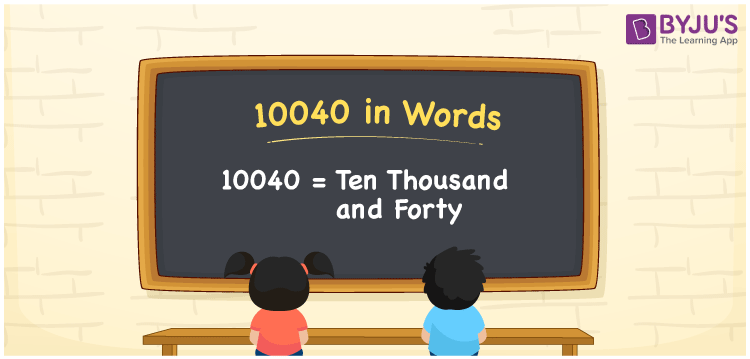# 10040 in Words

The number 10040 in words is written as “Ten Thousand and Forty”. If you purchased the mobile worth 10040, it can be written as “ I purchased the mobile worth Rs. Ten thousand and forty”. Since it expresses the value, the number 10040 is a cardinal number. The place value system is used to convert the number 10040 into words. Go through the below article to learn the place values of 10040 and its number name in detail.

 10040 in Words: Ten Thousand and Forty. Ten Thousand and Forty in Numerical Form: 10040.

## 10040 in English Words## How to Write 10040 in Words?

The below table depicts the place values of the number 10040:

 Ten-thousands Thousands Hundreds Tens Ones 1 0 0 4 0

The expanded form of 10040 is as follows:

= 1 × Ten thousand + 0 × Thousand + 0 × Hundred + 4 × Ten + 0 × One

= 1 × 10000 + 0 × 1000 + 0 × 100 + 4 × 10 + 0 × 1

= 10000 + 40

= 10040

= Ten thousand and forty

Hence, 10040 in words is ten thousand and forty.

10040 in words – Ten thousand and forty

Is 10040 an odd number? – No

Is 10040 an even number? – Yes

Is 10040 a perfect square number? – No

Is 10040 a perfect cube number? – No

Is 10040 a prime number? – No

Is 10040 a composite number? – Yes

## Frequently Asked Questions on 10040 in Words

Q1

### Write 10040 in words.

10040 in words is ten thousand and forty.

Q2

### Simplify 10000 + 40, and express it in words.

Simplifying 10000 + 40, we get 10040. Hence, 10040 in words is ten thousand and forty.

Q3

### Is 10040 a composite number?

Yes, 10040 is a composite number.

Test your Knowledge on 10040 in Words